# RD Sharma Solutions for Class 8 Maths Chapter 11 Time and Work

This chapter helps students understand the concepts based on time and work. In this chapter, we primarily discuss two points i.e., ‘finding the time required to complete a piece of work and finding the work done in a given period of time’. Solutions for such problems are derived in a step by step format by our experts to help students understand the concepts and come out with flying colours in their examinations. RD Sharma Maths solutions for Class 8  prepared by our experts is the best reference module one can use to secure good marks in their exams. Students can refer and easily download the pdf for free from the links given below.

Chapter 11- Time and Work contains one exercise and the RD Sharma Solutions present in this page provide solutions to the questions present in this exercise. Now, let us have a look at the concepts discussed in this chapter.

• Time and Work problems i.e., mainly (i. On finding the time required to complete a piece of work. ii. On finding the work done in a given period of time.)
• Pipes and cisterns problems.

## Download the pdf of RD Sharma Solutions For Class 8 Maths Chapter 11 Time and Work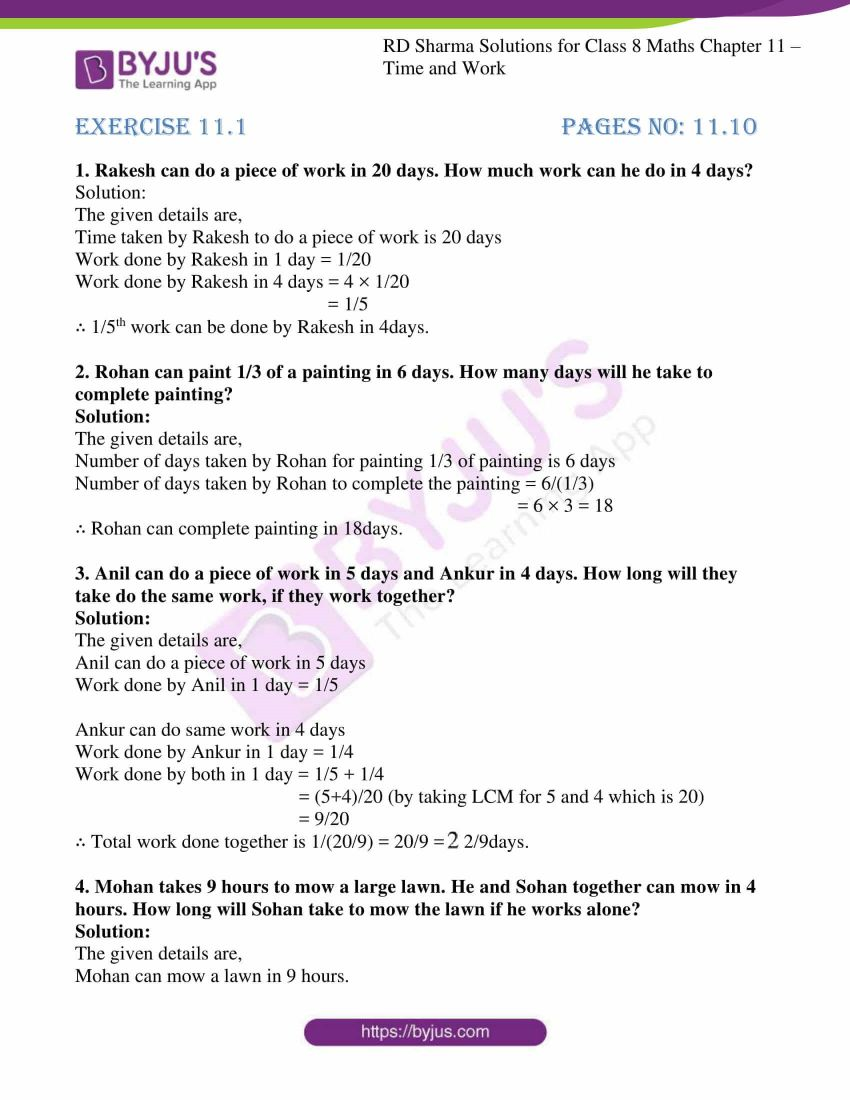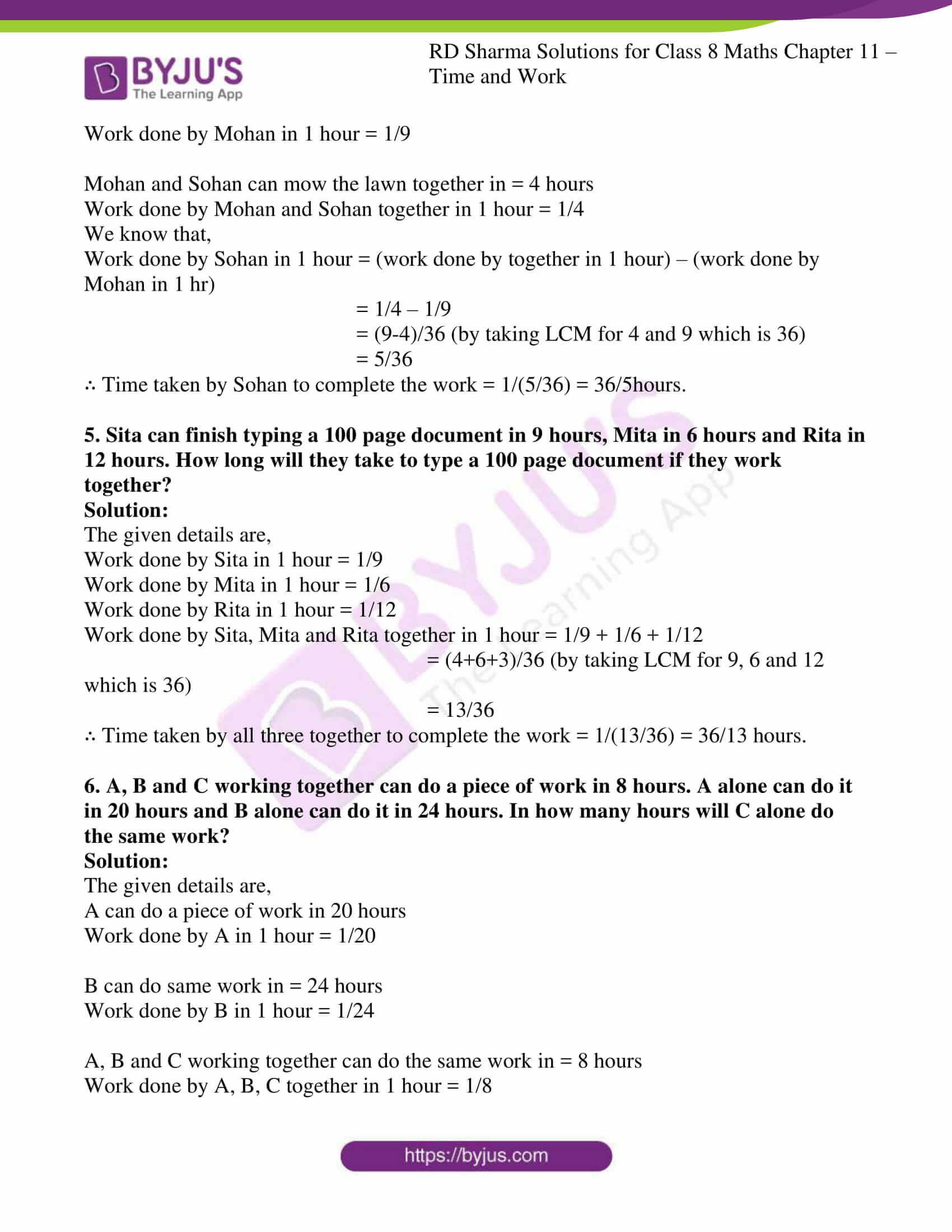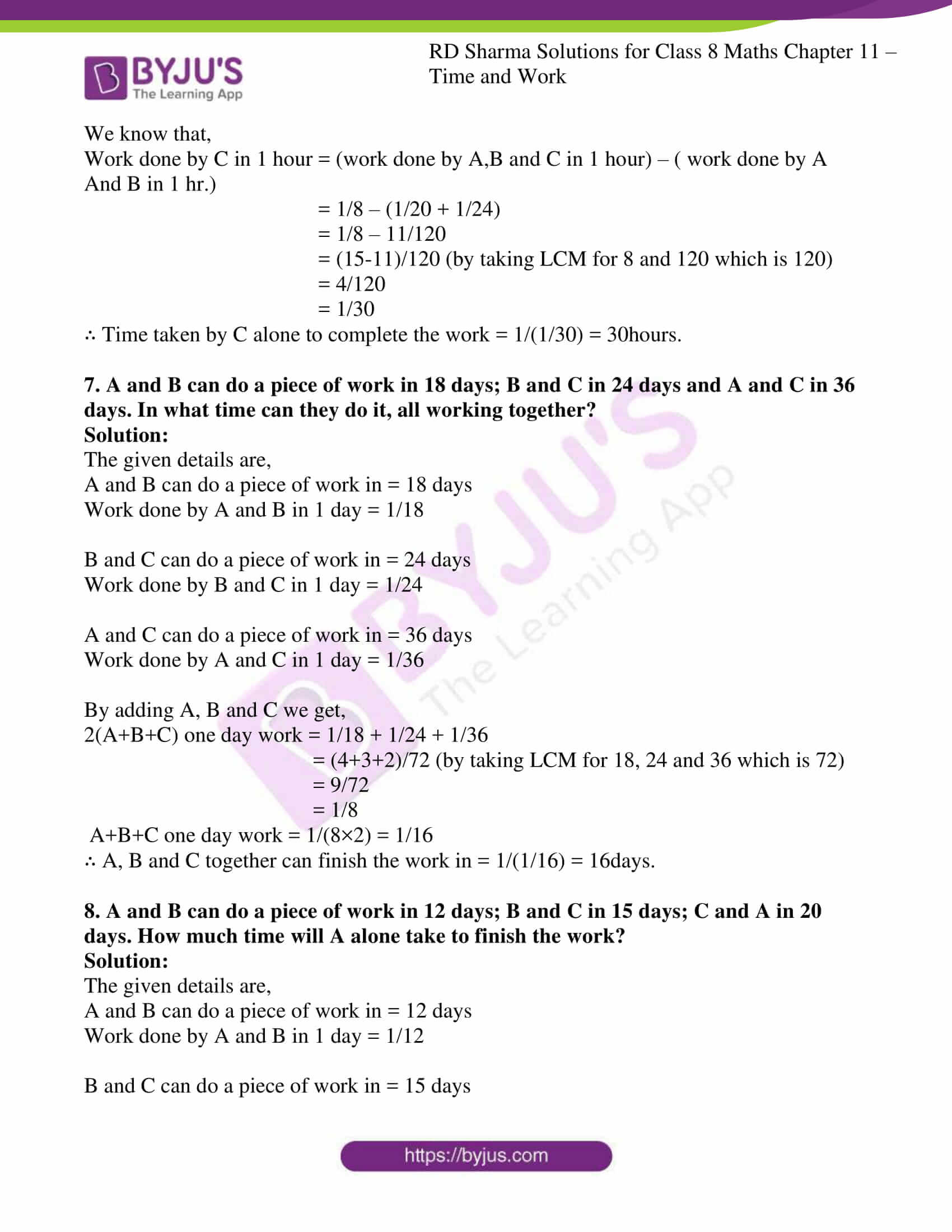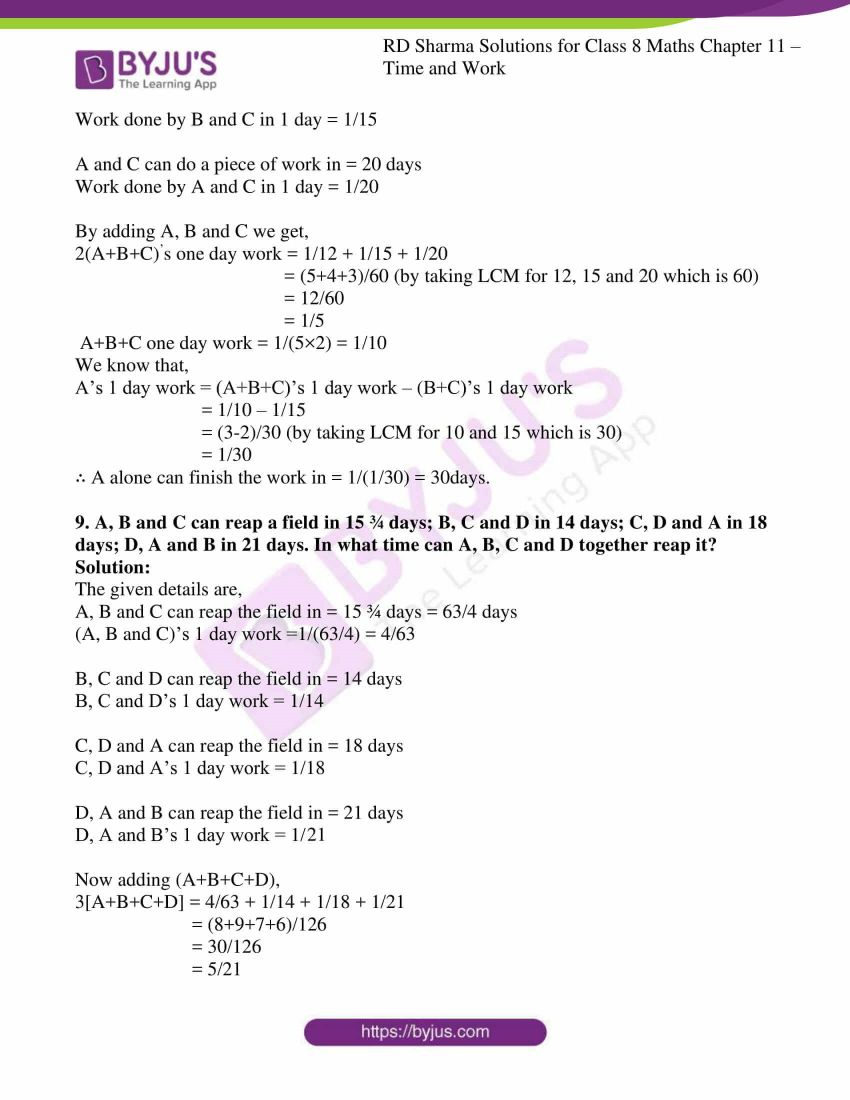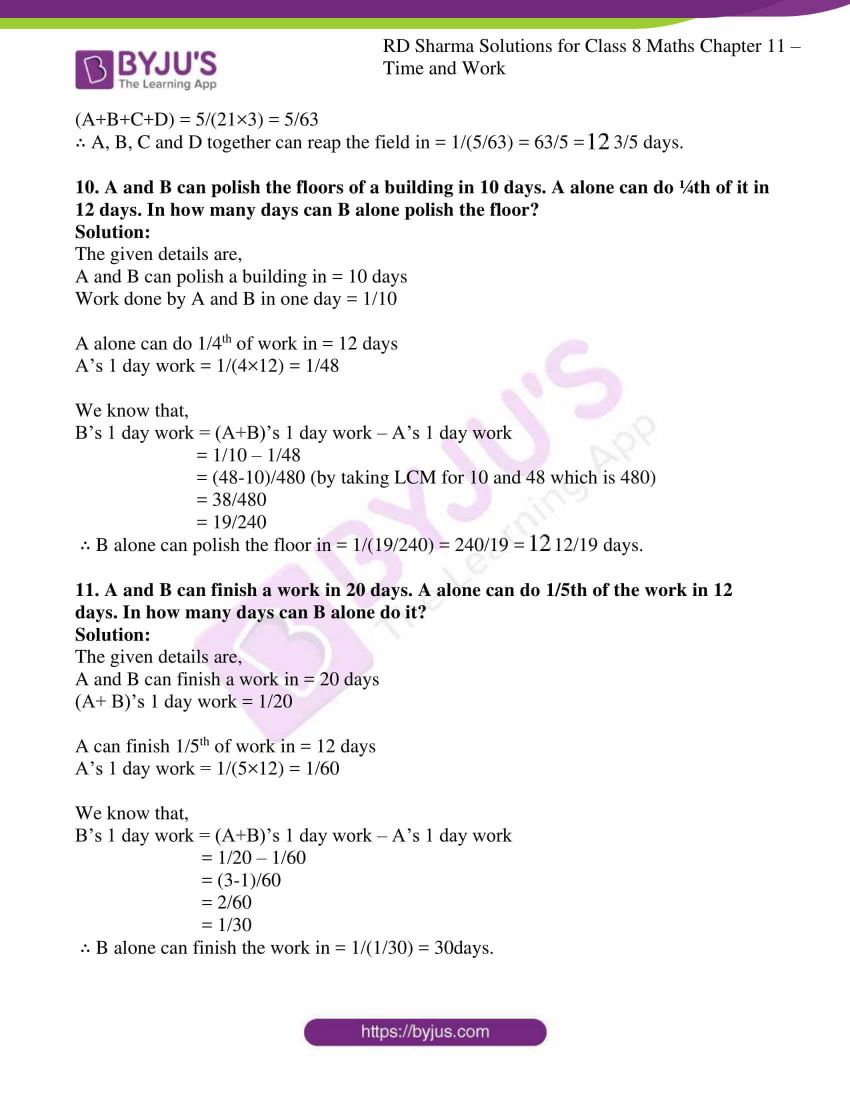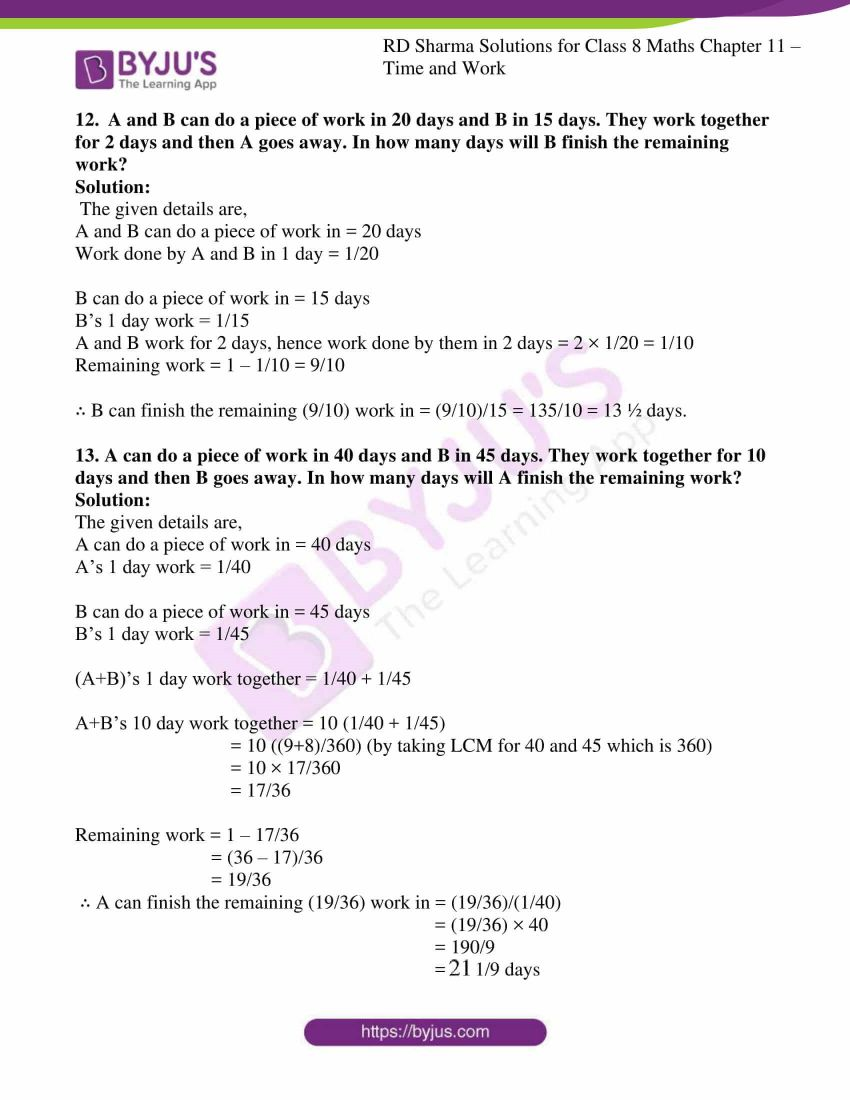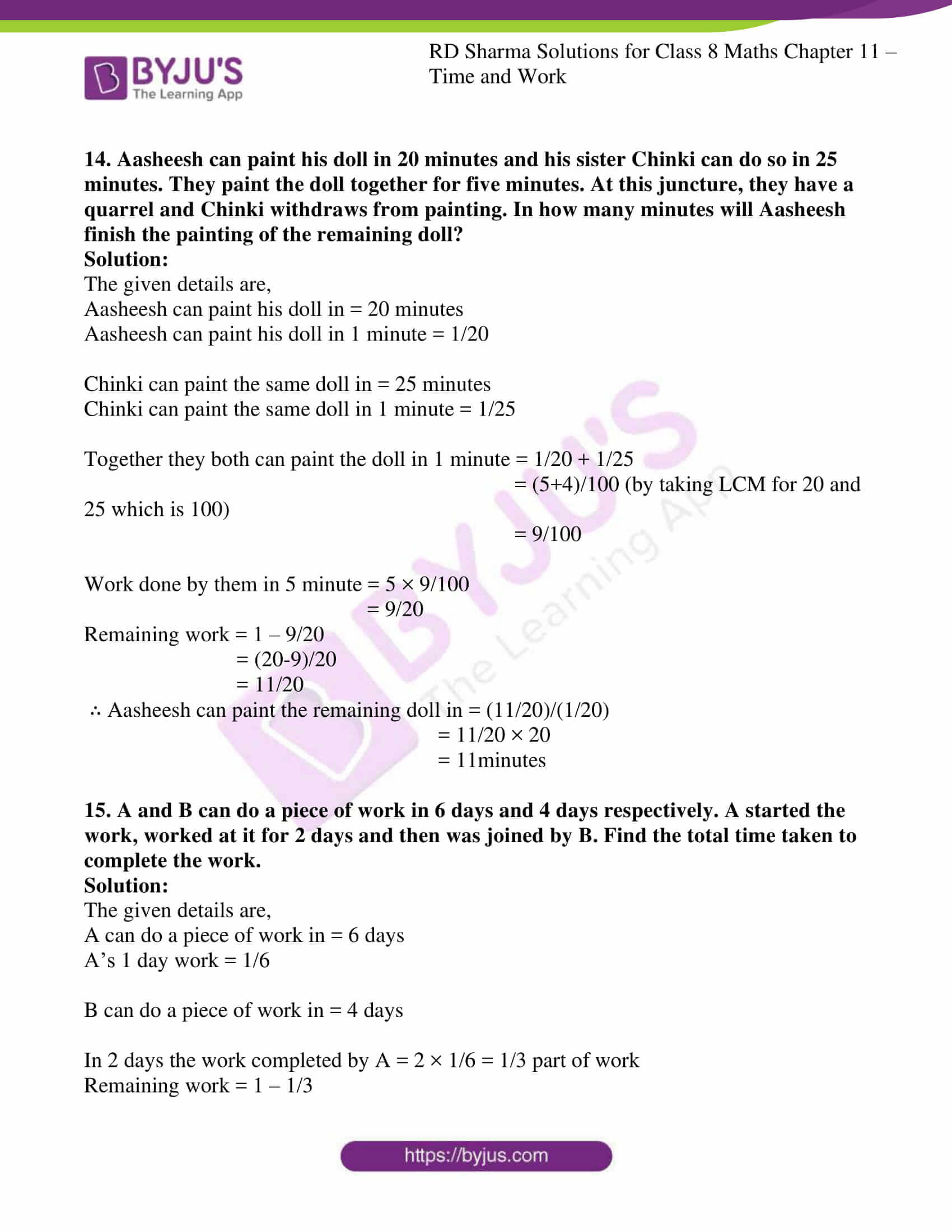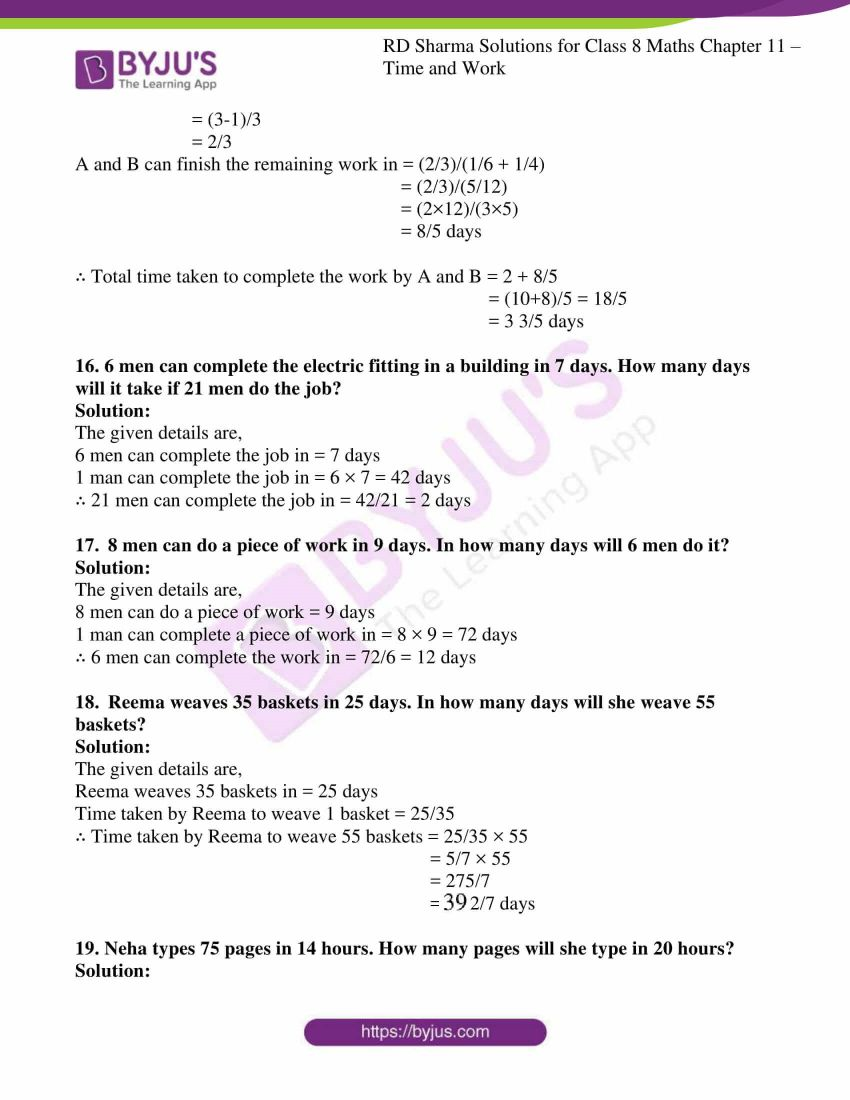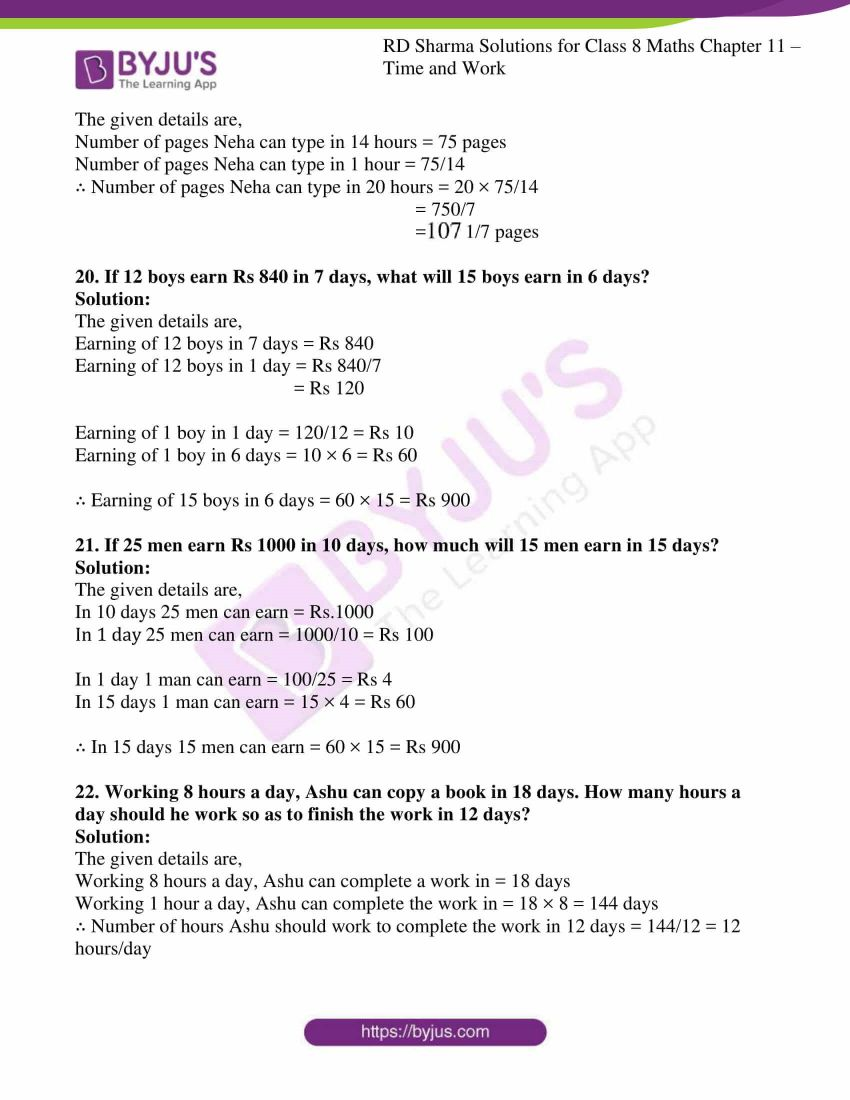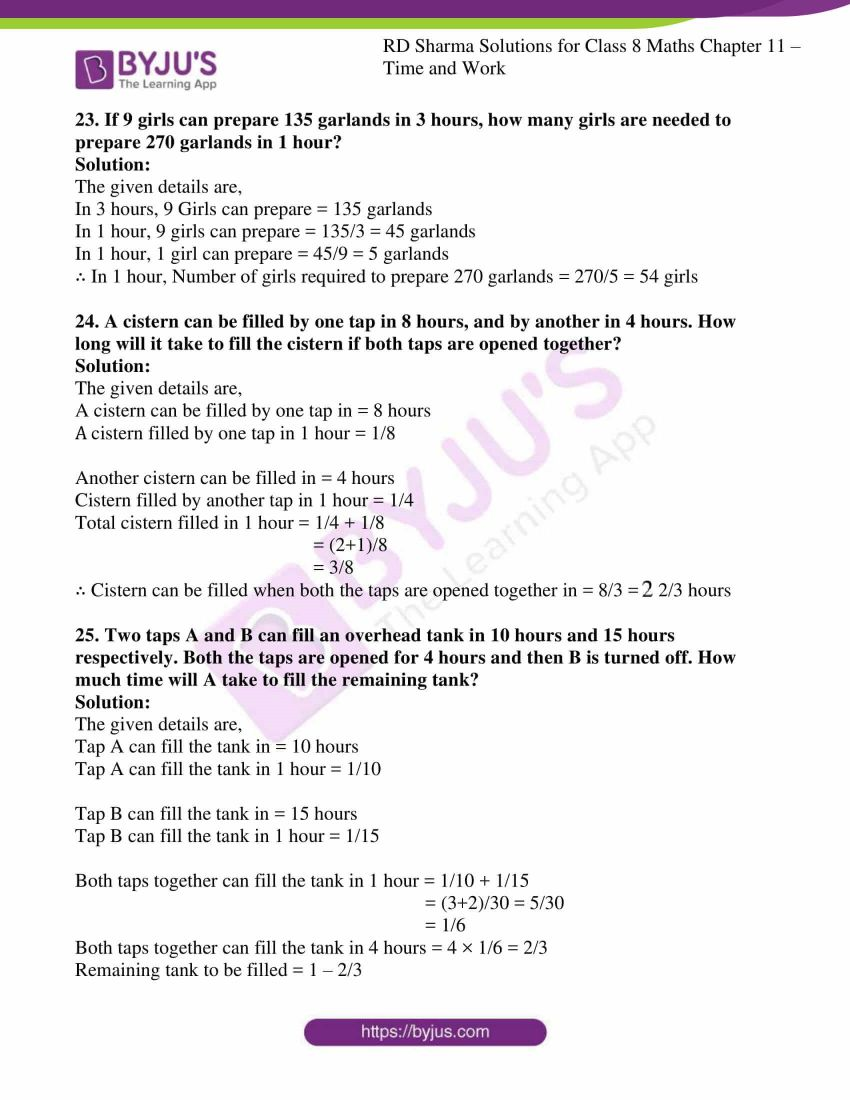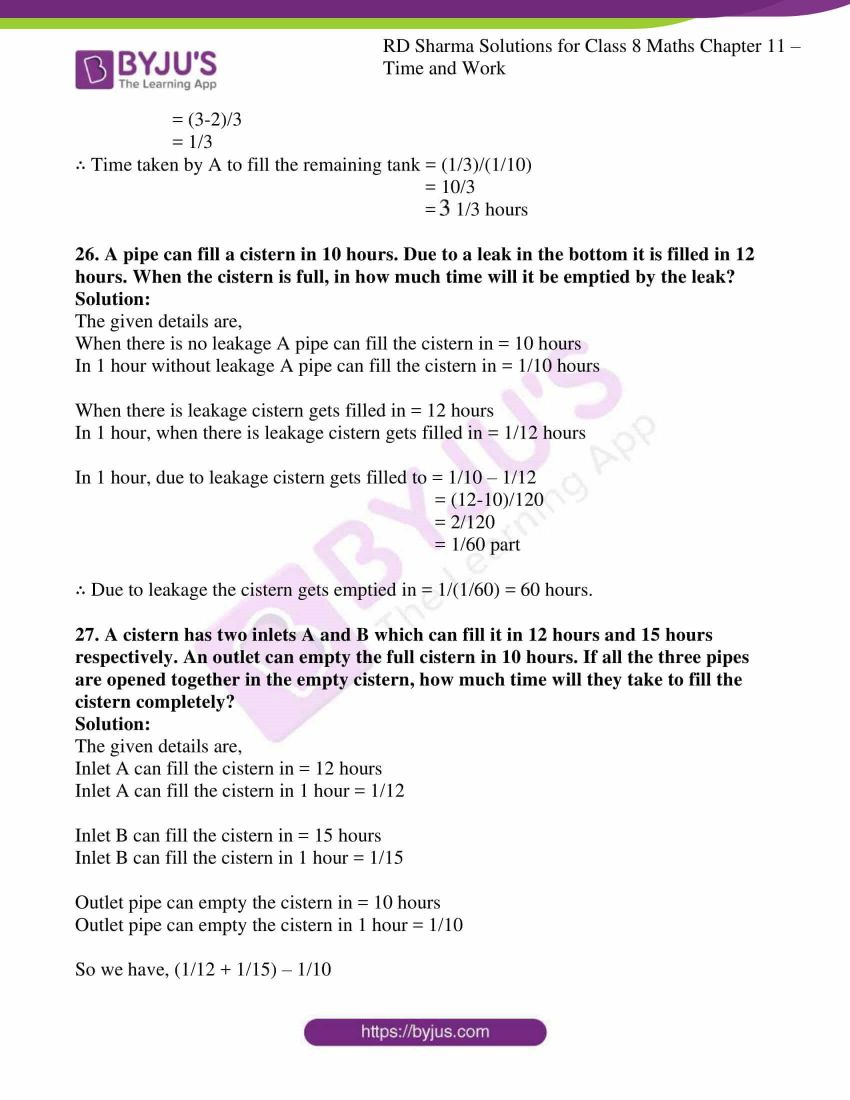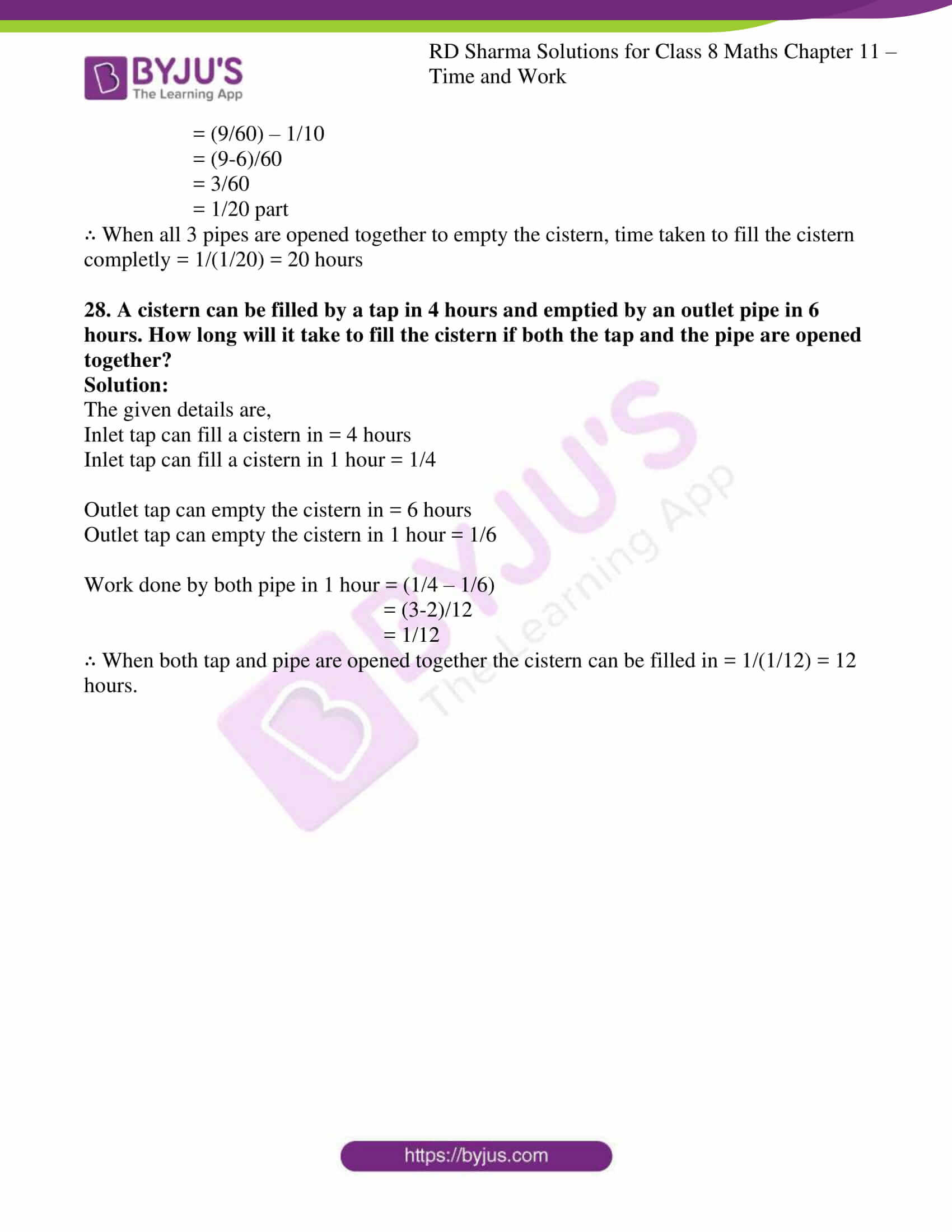### Access answers to Maths RD Sharma Solutions For Class 8 Chapter 11 Time and Work

1. Rakesh can do a piece of work in 20 days. How much work can he do in 4 days?

Solution:

The given details are,

Time taken by Rakesh to do a piece of work is 20 days

Work done by Rakesh in 1 day = 1/20

Work done by Rakesh in 4 days = 4 × 1/20

= 1/5

∴ 1/5th work can be done by Rakesh in 4days.

2. Rohan can paint 1/3 of a painting in 6 days. How many days will he take to complete painting?

Solution:

The given details are,

Number of days taken by Rohan for painting 1/3 of painting is 6 days

Number of days taken by Rohan to complete the painting = 6/(1/3)

= 6 × 3 = 18

∴ Rohan can complete painting in 18days.

3. Anil can do a piece of work in 5 days and Ankur in 4 days. How long will they take do the same work, if they work together?

Solution:

The given details are,

Anil can do a piece of work in 5 days

Work done by Anil in 1 day = 1/5

Ankur can do same work in 4 days

Work done by Ankur in 1 day = 1/4

Work done by both in 1 day = 1/5 + 1/4

= (5+4)/20 (by taking LCM for 5 and 4 which is 20)

= 9/20

∴ Total work done together is 1/(9/20) = 20/9 = $$2\frac{2}{9}$$ days.

4. Mohan takes 9 hours to mow a large lawn. He and Sohan together can mow in 4 hours. How long will Sohan take to mow the lawn if he works alone?

Solution:

The given details are,

Mohan can mow a lawn in 9 hours.

Work done by Mohan in 1 hour = 1/9

Mohan and Sohan can mow the lawn together in = 4 hours

Work done by Mohan and Sohan together in 1 hour = 1/4

We know that,

Work done by Sohan in 1 hour = (work done by together in 1 hour) – (work done by Mohan in 1 hr)

= 1/4 – 1/9

= (9-4)/36 (by taking LCM for 4 and 9 which is 36)

= 5/36

∴ Time taken by Sohan to complete the work = 1/(5/36) = 36/5hours.

5. Sita can finish typing a 100 page document in 9 hours, Mita in 6 hours and Rita in 12 hours. How long will they take to type a 100 page document if they work together?

Solution:

The given details are,

Work done by Sita in 1 hour = 1/9

Work done by Mita in 1 hour = 1/6

Work done by Rita in 1 hour = 1/12

Work done by Sita, Mita and Rita together in 1 hour = 1/9 + 1/6 + 1/12

= (4+6+3)/36 (by taking LCM for 9, 6 and 12 which is 36)

= 13/36

∴ Time taken by all three together to complete the work = 1/(13/36) = 36/13 hours.

6. A, B and C working together can do a piece of work in 8 hours. A alone can do it in 20 hours and B alone can do it in 24 hours. In how many hours will C alone do the same work?

Solution:

The given details are,

A can do a piece of work in 20 hours

Work done by A in 1 hour = 1/20

B can do same work in = 24 hours

Work done by B in 1 hour = 1/24

A, B and C working together can do the same work in = 8 hours

Work done by A, B, C together in 1 hour = 1/8

We know that,

Work done by C in 1 hour = (work done by A,B and C in 1 hour) – ( work done by A And B in 1 hr.)

= 1/8 – (1/20 + 1/24)

= 1/8 – 11/120

= (15-11)/120 (by taking LCM for 8 and 120 which is 120)

= 4/120

= 1/30

∴ Time taken by C alone to complete the work = 1/(1/30) = 30hours.

7. A and B can do a piece of work in 18 days; B and C in 24 days and A and C in 36 days. In what time can they do it, all working together?

Solution:

The given details are,

A and B can do a piece of work in = 18 days

Work done by A and B in 1 day = 1/18

B and C can do a piece of work in = 24 days

Work done by B and C in 1 day = 1/24

A and C can do a piece of work in = 36 days

Work done by A and C in 1 day = 1/36

By adding A, B and C we get,

2(A+B+C) one day work = 1/18 + 1/24 + 1/36

= (4+3+2)/72 (by taking LCM for 18, 24 and 36 which is 72)

= 9/72

= 1/8

A+B+C one day work = 1/(8×2) = 1/16

∴ A, B and C together can finish the work in = 1/(1/16) = 16days.

8. A and B can do a piece of work in 12 days; B and C in 15 days; C and A in 20 days. How much time will A alone take to finish the work?

Solution:

The given details are,

A and B can do a piece of work in = 12 days

Work done by A and B in 1 day = 1/12

B and C can do a piece of work in = 15 days

Work done by B and C in 1 day = 1/15

A and C can do a piece of work in = 20 days

Work done by A and C in 1 day = 1/20

By adding A, B and C we get,

2(A+B+C)s one day work = 1/12 + 1/15 + 1/20

= (5+4+3)/60 (by taking LCM for 12, 15 and 20 which is 60)

= 12/60

= 1/5

A+B+C one day work = 1/(5×2) = 1/10

We know that,

A’s 1 day work = (A+B+C)’s 1 day work – (B+C)’s 1 day work

= 1/10 – 1/15

= (3-2)/30 (by taking LCM for 10 and 15 which is 30)

= 1/30

∴ A alone can finish the work in = 1/(1/30) = 30days.

9. A, B and C can reap a field in 15 ¾ days; B, C and D in 14 days; C, D and A in 18 days; D, A and B in 21 days. In what time can A, B, C and D together reap it?

Solution:

The given details are,

A, B and C can reap the field in = 15 ¾ days = 63/4 days

(A, B and C)’s 1 day work =1/(63/4) = 4/63

B, C and D can reap the field in = 14 days

B, C and D’s 1 day work = 1/14

C, D and A can reap the field in = 18 days

C, D and A’s 1 day work = 1/18

D, A and B can reap the field in = 21 days

D, A and B’s 1 day work = 1/21

3[A+B+C+D] = 4/63 + 1/14 + 1/18 + 1/21

= (8+9+7+6)/126

= 30/126

= 5/21

(A+B+C+D) = 5/(21×3) = 5/63

∴ A, B, C and D together can reap the field in = 1/(5/63) = 63/5 = $$12\frac{3}{5}$$ days.

10. A and B can polish the floors of a building in 10 days. A alone can do ¼th of it in 12 days. In how many days can B alone polish the floor?

Solution:

The given details are,

A and B can polish a building in = 10 days

Work done by A and B in one day = 1/10

A alone can do 1/4th of work in = 12 days

A’s 1 day work = 1/(4×12) = 1/48

We know that,

B’s 1 day work = (A+B)’s 1 day work – A’s 1 day work

= 1/10 – 1/48

= (48-10)/480 (by taking LCM for 10 and 48 which is 480)

= 38/480

= 19/240

∴ B alone can polish the floor in = 1/(19/240) = 240/19 = $$12\frac{12}{19}$$ days.

11. A and B can finish a work in 20 days. A alone can do 1/5th of the work in 12 days. In how many days can B alone do it?

Solution:

The given details are,

A and B can finish a work in = 20 days

(A+ B)’s 1 day work = 1/20

A can finish 1/5th of work in = 12 days

A’s 1 day work = 1/(5×12) = 1/60

We know that,

B’s 1 day work = (A+B)’s 1 day work – A’s 1 day work

= 1/20 – 1/60

= (3-1)/60

= 2/60

= 1/30

∴ B alone can finish the work in = 1/(1/30) = 30days.

12. A and B can do a piece of work in 20 days and B in 15 days. They work together for 2 days and then A goes away. In how many days will B finish the remaining work?

Solution:

The given details are,

A and B can do a piece of work in = 20 days

Work done by A and B in 1 day = 1/20

B can do a piece of work in = 15 days

B’s 1 day work = 1/15

A and B work for 2 days, hence work done by them in 2 days = 2 × 1/20 = 1/10

Remaining work = 1 – 1/10 = 9/10

∴ B can finish the remaining (9/10) work in = (9/10)/15 = 135/10 = 13 ½ days.

13. A can do a piece of work in 40 days and B in 45 days. They work together for 10 days and then B goes away. In how many days will A finish the remaining work?

Solution:

The given details are,

A can do a piece of work in = 40 days

A’s 1 day work = 1/40

B can do a piece of work in = 45 days

B’s 1 day work = 1/45

(A+B)’s 1 day work together = 1/40 + 1/45

A+B’s 10 day work together = 10 (1/40 + 1/45)

= 10 ((9+8)/360) (by taking LCM for 40 and 45 which is 360)

= 10 × 17/360

= 17/36

Remaining work = 1 – 17/36

= (36 – 17)/36

= 19/36

∴ A can finish the remaining (19/36) work in = (19/36)/(1/40)

= (19/36) × 40

= 190/9

= $$21\frac{1}{9}$$ days

14. Aasheesh can paint his doll in 20 minutes and his sister Chinki can do so in 25 minutes. They paint the doll together for five minutes. At this juncture, they have a quarrel and Chinki withdraws from painting. In how many minutes will Aasheesh finish the painting of the remaining doll?

Solution:

The given details are,

Aasheesh can paint his doll in = 20 minutes

Aasheesh can paint his doll in 1 minute = 1/20

Chinki can paint the same doll in = 25 minutes

Chinki can paint the same doll in 1 minute = 1/25

Together they both can paint the doll in 1 minute = 1/20 + 1/25

= (5+4)/100 (by taking LCM for 20 and 25 which is 100)

= 9/100

Work done by them in 5 minute = 5 × 9/100

= 9/20

Remaining work = 1 – 9/20

= (20-9)/20

= 11/20

∴ Aasheesh can paint the remaining doll in = (11/20)/(1/20)

= 11/20 × 20

= 11minutes

15. A and B can do a piece of work in 6 days and 4 days respectively. A started the work, worked at it for 2 days and then was joined by B. Find the total time taken to complete the work.

Solution:

The given details are,

A can do a piece of work in = 6 days

A’s 1 day work = 1/6

B can do a piece of work in = 4 days

In 2 days the work completed by A = 2 × 1/6 = 1/3 part of work

Remaining work = 1 – 1/3

= (3-1)/3

= 2/3

A and B can finish the remaining work in = (2/3)/(1/6 + 1/4)

= (2/3)/(5/12)

= (2×12)/(3×5)

= 8/5 days

∴ Total time taken to complete the work by A and B = 2 + 8/5

= (10+8)/5 = 18/5

= 3 3/5 days

16. 6 men can complete the electric fitting in a building in 7 days. How many days will it take if 21 men do the job?

Solution:

The given details are,

6 men can complete the job in = 7 days

1 man can complete the job in = 6 × 7 = 42 days

∴ 21 men can complete the job in = 42/21 = 2 days

17. 8 men can do a piece of work in 9 days. In how many days will 6 men do it?

Solution:

The given details are,

8 men can do a piece of work = 9 days

1 man can complete a piece of work in = 8 × 9 = 72 days

∴ 6 men can complete the work in = 72/6 = 12 days

18. Reema weaves 35 baskets in 25 days. In how many days will she weave 55 baskets?

Solution:

The given details are,

Reema weaves 35 baskets in = 25 days

Time taken by Reema to weave 1 basket = 25/35

∴ Time taken by Reema to weave 55 baskets = 25/35 × 55

= 5/7 × 55

= 275/7

= $$39\frac{2}{7}$$ days

19. Neha types 75 pages in 14 hours. How many pages will she type in 20 hours?

Solution:

The given details are,

Number of pages Neha can type in 14 hours = 75 pages

Number of pages Neha can type in 1 hour = 75/14

∴ Number of pages Neha can type in 20 hours = 20 × 75/14

= 750/7

= $$107\frac{1}{7}$$ pages

20. If 12 boys earn Rs 840 in 7 days, what will 15 boys earn in 6 days?

Solution:

The given details are,

Earning of 12 boys in 7 days = Rs 840

Earning of 12 boys in 1 day = Rs 840/7

= Rs 120

Earning of 1 boy in 1 day = 120/12 = Rs 10

Earning of 1 boy in 6 days = 10 × 6 = Rs 60

∴ Earning of 15 boys in 6 days = 60 × 15 = Rs 900

21. If 25 men earn Rs 1000 in 10 days, how much will 15 men earn in 15 days?

Solution:

The given details are,

In 10 days 25 men can earn = Rs.1000

In 1 day 25 men can earn = 1000/10 = Rs 100

In 1 day 1 man can earn = 100/25 = Rs 4

In 15 days 1 man can earn = 15 × 4 = Rs 60

∴ In 15 days 15 men can earn = 60 × 15 = Rs 900

22. Working 8 hours a day, Ashu can copy a book in 18 days. How many hours a day should he work so as to finish the work in 12 days?

Solution:

The given details are,

Working 8 hours a day, Ashu can complete a work in = 18 days

Working 1 hour a day, Ashu can complete the work in = 18 × 8 = 144 days

∴ Number of hours Ashu should work to complete the work in 12 days = 144/12 = 12 hours/day

23. If 9 girls can prepare 135 garlands in 3 hours, how many girls are needed to prepare 270 garlands in 1 hour?

Solution:

The given details are,

In 3 hours, 9 Girls can prepare = 135 garlands

In 1 hour, 9 girls can prepare = 135/3 = 45 garlands

In 1 hour, 1 girl can prepare = 45/9 = 5 garlands

∴ In 1 hour, Number of girls required to prepare 270 garlands = 270/5 = 54 girls

24. A cistern can be filled by one tap in 8 hours, and by another in 4 hours. How long will it take to fill the cistern if both taps are opened together?

Solution:

The given details are,

A cistern can be filled by one tap in = 8 hours

A cistern filled by one tap in 1 hour = 1/8

Another cistern can be filled in = 4 hours

Cistern filled by another tap in 1 hour = 1/4

Total cistern filled in 1 hour = 1/4 + 1/8

= (2+1)/8

= 3/8

∴ Cistern can be filled when both the taps are opened together in = 8/3 = $$2\frac{2}{3}$$ hours

25. Two taps A and B can fill an overhead tank in 10 hours and 15 hours respectively. Both the taps are opened for 4 hours and then B is turned off. How much time will A take to fill the remaining tank?

Solution:

The given details are,

Tap A can fill the tank in = 10 hours

Tap A can fill the tank in 1 hour = 1/10

Tap B can fill the tank in = 15 hours

Tap B can fill the tank in 1 hour = 1/15

Both taps together can fill the tank in 1 hour = 1/10 + 1/15

= (3+2)/30 = 5/30

= 1/6

Both taps together can fill the tank in 4 hours = 4 × 1/6 = 2/3

Remaining tank to be filled = 1 – 2/3

= (3-2)/3

= 1/3

∴ Time taken by A to fill the remaining tank = (1/3)/(1/10)

= 10/3

= $$3\frac{1}{3}$$ hours

26. A pipe can fill a cistern in 10 hours. Due to a leak in the bottom it is filled in 12 hours. When the cistern is full, in how much time will it be emptied by the leak?

Solution:

The given details are,

When there is no leakage A pipe can fill the cistern in = 10 hours

In 1 hour without leakage A pipe can fill the cistern in = 1/10 hours

When there is leakage cistern gets filled in = 12 hours

In 1 hour, when there is leakage cistern gets filled in = 1/12 hours

In 1 hour, due to leakage cistern gets filled to = 1/10 – 1/12

= (12-10)/120

= 2/120

= 1/60 part

∴ Due to leakage the cistern gets emptied in = 1/(1/60) = 60 hours.

27. A cistern has two inlets A and B which can fill it in 12 hours and 15 hours respectively. An outlet can empty the full cistern in 10 hours. If all the three pipes are opened together in the empty cistern, how much time will they take to fill the cistern completely?

Solution:

The given details are,

Inlet A can fill the cistern in = 12 hours

Inlet A can fill the cistern in 1 hour = 1/12

Inlet B can fill the cistern in = 15 hours

Inlet B can fill the cistern in 1 hour = 1/15

Outlet pipe can empty the cistern in = 10 hours

Outlet pipe can empty the cistern in 1 hour = 1/10

So we have, (1/12 + 1/15) – 1/10

= (9/60) – 1/10

= (9-6)/60

= 3/60

= 1/20 part

∴ When all 3 pipes are opened together to empty the cistern, time taken to fill the cistern completly = 1/(1/20) = 20 hours

28. A cistern can be filled by a tap in 4 hours and emptied by an outlet pipe in 6 hours. How long will it take to fill the cistern if both the tap and the pipe are opened together?

Solution:

The given details are,

Inlet tap can fill a cistern in = 4 hours

Inlet tap can fill a cistern in 1 hour = 1/4

Outlet tap can empty the cistern in = 6 hours

Outlet tap can empty the cistern in 1 hour = 1/6

Work done by both pipe in 1 hour = (1/4 – 1/6)

= (3-2)/12

= 1/12

∴ When both tap and pipe are opened together the cistern can be filled in = 1/(1/12) = 12 hours.